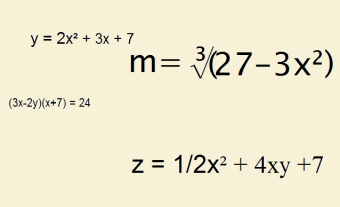# Constants and Variables: A Simple Introduction to Algebra

1. Home
2. /
3. Mathematics
4. /
5. Constants and Variables: A...Please bear with me on this article. You see, I am a chemist, not a mathematician. Yet, as an individual who struggled with the concepts behind algebra (yet I grasped it soon enough to ace it), I can understand how others – intelligent individuals – can find algebra disconcerting. What are constants and variables?

## Two Basic Participants – Constants and Variables

There are two primary participants in algebra – variables (which change) and constants (which do not change). Constants are specific numbers that never change. 27 is always 27. 43-1/4 is always 43-1/4. It never changes; it is constant.

So let’s consider your age. Your age changes! This year you may be 16. Next year, you will be 17. Age is variable. Let’s write an equation.Pick a number. Let’s say you are 14 this year (2016). You get older as time passes. Yet, ten years from now (2026) you can still honestly say you were 14 in 2016. As each year passes, your age goes up by 1. In 2017 you are 15. In 2018 you are 16. In 2019 you are 17.

Let us write an equation that spells all of this out. We have to create a variable that stands for your age. We’ll call your age A. The year we’ll call Y. In 2017, Y = 2017. In 2018, Y = 2018, and so on. Then, in terms of constants and variables,

A = 14 + (Y – 2016) [Equation 1]

This equation tell us your age is 14 plus the current year minus the year in which you were 14. Let’s say it is the year 2023. How old are you then?

A = 14 + (2023 – 2016)

Now 2023 – 2016 = 7. Therefore,

A = 14 + 7 = 21

You are 21 years old in 2023. This makes sense, doesn’t it? Count it out on your fingers, if you doubt it.## Introduction to Algebra – Equation Simplification

The equation listed above as Equation 1 is correct as written. But you believe your equation can be simplified. That is,

A = 14 + (Y – 2016)

Can be written more simply. How so? Well both 14 and 2016 are constants. They never change. So you should be able to combine them.

14 – 2016 = –2002

So your first algebra equation (expressed in constants and variables) can be written more simply,

A = Y – 2002 [Equation 2]

## In Conclusion

But you’re just a little nervous. Is this equation correct? It seems it should be. Let’s check.

14 = 2016 – 2002

This is correct! Congratulations. You have succeeded in writing – and simplifying – your very first algebra equation. Oh, one last thing… In beginning algebra courses, the variable names chosen are often x and y, though any name will do. In that case, our equation may be written x = y – 2002. Too, it is typical of an early course to see y in terms of x, with y on the left. If that is the case, we write: y = x + 2002.

Note: You might also enjoy Algebra for Beginners: Student Perspective

### One thought on “Constants and Variables: A Simple Introduction to Algebra”

• I enjoyed simple algebra, right up to quadratic equations (not that I could do one now!) but never took it any further. I suppose, now that I have finished my main studying project, I could take up maths again. It would certainly help keep the old brain active!